Latest Banking jobs   »   Quantitative Aptitude Quiz For IBPS PO...

# Quantitative Aptitude Quiz For IBPS PO Mains 2022- 6th January

Directions (1-4): The following questions are accompanied by two statements I and II. You have to determine which statements(s) is/are sufficient/necessary to answer the questions.

Q1. Find the sum of present age of X & Z?
I. Four years ago, average age of X, Y and Z was 16 years while age of X, Y and Z is in arithmetic progression.
II. Average of present age of X and Z is same as present age of Y while Y is four years younger than Z.
(a) Statement I alone is sufficient to answer the question but statement II alone is not sufficient to answer the question.
(b) Statement II alone is sufficient to answer the question but statement I alone is not sufficient to answer the question.
(c) Both the statements taken together are necessary to answer the questions, but neither of the statements alone is sufficient to answer the question.
(d) Either statement I or statement II by itself is sufficient to answer the question.
(e) Statements I and II taken together are not sufficient to answer the question.

Q2. Is Rahul meet Prabhat at half of the distance on a circular track (48km) if they both started to move at same time in same direction?
I. Speed of Prabhat is 24km/hr more than speed of Rahul.
II. Prabhat’s speed is 200% more than that of Rahul
(a) Statement I alone is sufficient to answer the question but statement II alone is not sufficient to answer the question.
(b) Either statement I or statement II by itself is sufficient to answer the question.
(c) Both the statements taken together are necessary to answer the questions, but neither of the statements alone is sufficient to answer the question.
(d) Statement II alone is sufficient to answer the question but statement I alone is not sufficient to answer the question.
(e) Statements I and II taken together are not sufficient to answer the question.

Q3. Find number of yellow balls in a box which contains 3 black balls and 7 red and yellow balls.
I. Probability of selecting two yellow balls from the box is 2/15
II. Probability of selecting one red and black ball is ⅕.
(a) Statement I alone is sufficient to answer the question but statement II alone is not sufficient to answer the question.
(b) Statement II alone is sufficient to answer the question but statement I alone is not sufficient to answer the question.
(c) Both the statements taken together are necessary to answer the questions, but neither of the statements alone is sufficient to answer the question.
(d) Either statement I or statement II by itself is sufficient to answer the question.
(e) Statements I and II taken together are not sufficient to answer the question.

Q4. Find number of pens sold by retailer if he earns total 37.5% profit on selling some pens and some pencils.
I. Ratio between pen sold to pencil sold is 2 : 3 while ratio between cost price of pen to pencil is 3 : 2.
II. On selling a pen and a pencil, he earns 25% and 50% profit respectively.
(a) Both the statements taken together are necessary to answer the questions, but neither of the statements alone is sufficient to answer the question.
(b) Statements I and II taken together are not sufficient to answer the question.
(c) Statement II alone is sufficient to answer the question but statement I alone is not sufficient to answer the question.
(d) Statement I alone is sufficient to answer the question but statement II alone is not sufficient to answer the question.
(e) Either statement I or statement II by itself is sufficient to answer the question.

Q5. Veer, Satish and Neeraj started a business together. They invested in the ratio 2 : 3 : 4. Neeraj left the business after working for 6 months. Veer and Satish worked for ‘x’ and ‘y’ months respectively.
Quantity I: Value of ‘x’, If profit share of all three is equal.
Quantity II: Value of ‘y’, if profit share of Veer, Satish and Neeraj is in the ratio 2 : 3 : 2
(a) Quantity I > Quantity II
(b) Quantity I < Quantity II
(c) Quantity I ≥ Quantity II
(d) Quantity I ≤ Quantity II
(e) Quantity I = Quantity II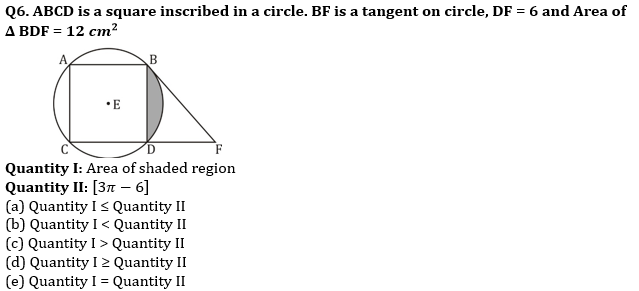Q7. Quantity I: Profit percentage on selling all the three articles.
The cost price of three articles are in ratio 3 : 4 : 5. The shopkeeper marks up the price of these articles by 100%, 50% and 20% respectively and then offers a discount of 20% on each article.
Quantity II: Profit percentage on selling second book.
Atul bought a book for Rs. 750 and sold it at 25% profit. With that amount he obtained second book and sold it at 20% discount.
(a) Quantity I > Quantity II
(b) Quantity I < Quantity II
(c) Quantity I≥ Quantity II
(d) Quantity I ≤ Quantity II
(e) Quantity I = Quantity II

Q8. Quantity I: Surface area of one of the parts.
A right circular cylinder of radius 4 cm and height 18 cm is melted and recast into a sphere. The sphere is then cut down into two equal parts by a plane.
Quantity II: Area of original rectangle plot.
A square plot is placed adjacent to a rectangular plot having breadth equal to side of the square. Ratio of length to breadth of rectangular plot is 7 : 3 and perimeter of newly formed rectangular plot is 104 cm.
(a) Quantity I> Quantity II
(b) Quantity I< Quantity II
(c) Quantity I≥ Quantity II
(d) Quantity I ≤ Quantity II
(e) Quantity I = Quantity II

Directions (9-10): In the given questions, two quantities are given, one as Quantity I and another as Quantity II. You have to determine relationship between two quantities and choose the appropriate option.

Q9. There are three vessel A, B and C. Vessel A contains mixture of milk and water in ratio 3 : 1. Vessel B contains 20 litres of pure water while vessel C contains 30 litres of pure milk. Half of the content of vessel A is first poured into vessel B. Then content of vessel B is poured into vessel C and finally contents of vessel C is poured into vessel A. The final ratio of milk and water in vessel A is 9 : 4.
Quantity I: Initial quantity of mixture in vessel A.
Quantity II: 80 litres.
(a) Quantity I > Quantity II
(b) Quantity I < Quantity II
(c) Quantity I ≥ Quantity II
(d) Quantity I ≤ Quantity II
(e) Quantity I = Quantity II or No relation

Q10. 20 men can complete a work in 12 days. 5 women are as efficient as 3 men. 4 men and 10 women started working and they already worked for 8 days.
Quantity I: Additional number of women required to complete the remaining work in 10 days.
Quantity II: Additional number of men required to complete the remaining work in either 8 or less than 8 days.
(a) Quantity I > Quantity II
(b) Quantity I < Quantity II
(c) Quantity I ≥ Quantity II
(d) Quantity I ≤ Quantity II
(e) Quantity I = Quantity II or No relation

Solutions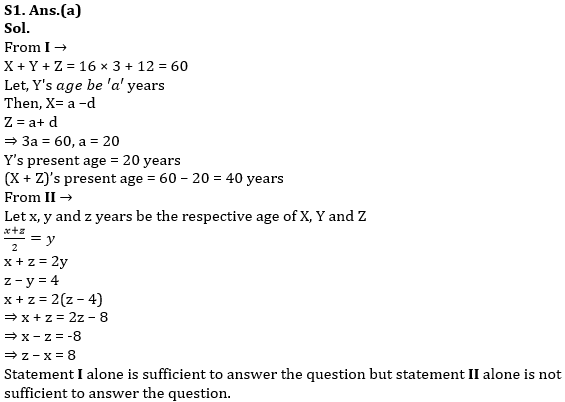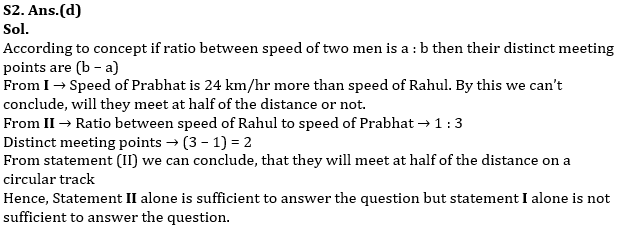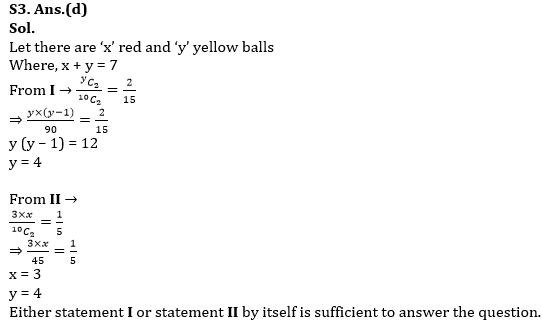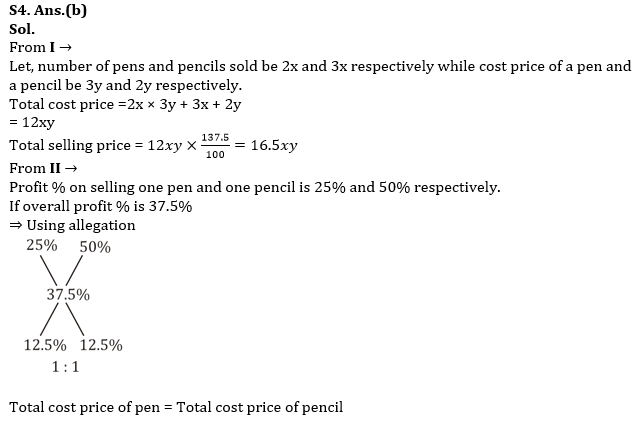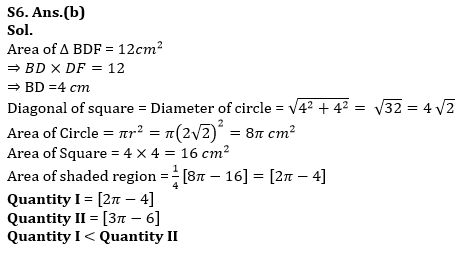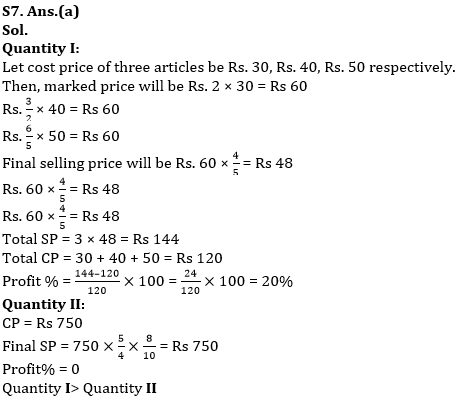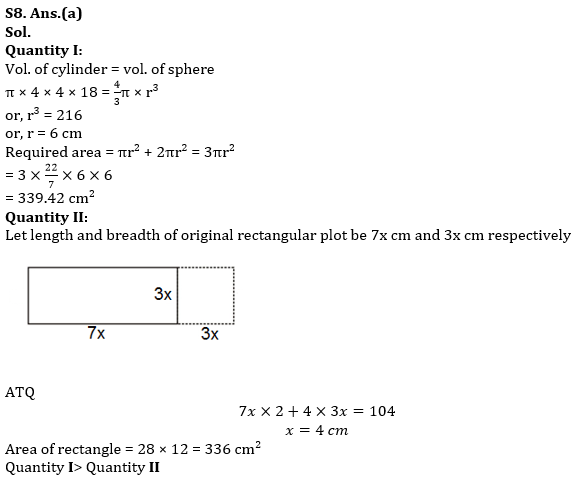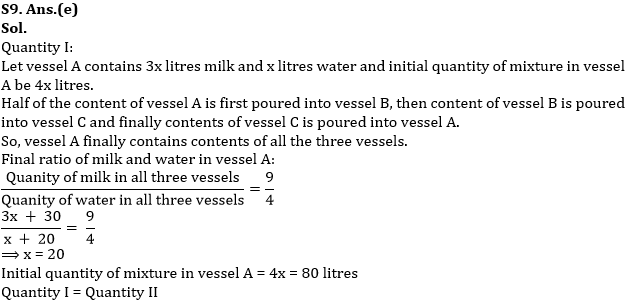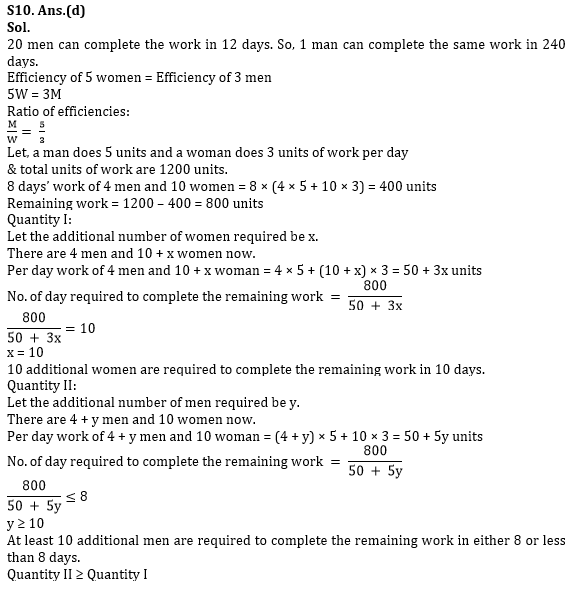#### Congratulations!Union Budget 2023-24: Free PDF